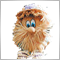Moderator
270651

This thread discusses MQL5 code examples. There will be examples of how to get data from indicators, how to program advisors ... in general, any questions from beginner to the MQL5 language.

If you are just starting to get acquainted with the MetaTrader 5 terminal, then I can recommend the following threads:Moderator
270651

This is the question I was asked recently: how to get data from the iBearsPower indicator?

Before showing the code, I’ll start with the main points of working with indicators in MQL5 Expert Advisors:

Step 1: at the global program level, we declare a variable - the handle of the iBearsPower indicator will be stored in this variable.

Step 2: in OnInit () we create the iBearsPower indicator and write the handle to the variable. Note: the indicator is created ONCE in OnInit ().

Step 3: in OnTick () we get data from the indicator

An example is in CodeBase in the code MySystem .

Step 1:

```double ExtStopLoss=0.0;
double ExtTakeProfit=0.0;

int    handle_iBullsPower;          // variable for storing the handle of the iBullsPower indicator
int    handle_iBearsPower;          // variable for storing the handle of the iBearsPower indicator

//+------------------------------------------------------------------+
//| Expert initialization function                                   |
//+------------------------------------------------------------------+
int OnInit()```

Step 2:

```     }
//--- create handle of the indicator iBearsPower
handle_iBearsPower=iBearsPower(m_symbol.Name(),Period(),Inp_ma_period);
//--- if the handle is not created
if(handle_iBearsPower==INVALID_HANDLE)
{
//--- tell about the failure and output the error code
PrintFormat("Failed to create handle of the iBearsPower indicator for the symbol %s/%s, error code %d",
m_symbol.Name(),
EnumToString(Period()),
GetLastError());
//--- the indicator is stopped early
return(INIT_FAILED);
}
//---
return(INIT_SUCCEEDED);
}
//+------------------------------------------------------------------+
//| Expert deinitialization function                                 |
//+------------------------------------------------------------------+
void OnDeinit(const int reason)```

Step 3:

```   double bulls[];
ArraySetAsSeries(bulls,true);
double bears[];
ArraySetAsSeries(bears,true);
if(!iBullsPowerGetArray(InpBarCurrent,2,bulls) || !iBearsPowerGetArray(InpBarCurrent,2,bears))
{
PrevBars=0;
return;

}```

Function iBearsPowerGetArray: is used CopyBuffer

```//+------------------------------------------------------------------+
//| Get value of buffers for the iBearsPower in the array            |
//+------------------------------------------------------------------+
bool iBearsPowerGetArray(const int start_pos,const int count,double &arr_buffer[])
{
//---
bool result=true;
if(!ArrayIsDynamic(arr_buffer))
{
Print("This a no dynamic array!");
return(false);
}
ArrayFree(arr_buffer);
int       buffer_num=0;          // indicator buffer number
//--- reset error code
ResetLastError();
//--- fill a part of the iBearsPower array with values from the indicator buffer that has 0 index
int copied=CopyBuffer(handle_iBearsPower,buffer_num,start_pos,count,arr_buffer);
if(copied!=count)
{
//--- if the copying fails, tell the error code
PrintFormat("Failed to copy data from the iBearsPower indicator, error code %d",GetLastError());
//--- quit with zero result - it means that the indicator is considered as not calculated
return(false);
}
return(result);
}```Moderator
270651

How to receive data from indicators from several symbols.

Everything is very simple! First you need to specify the names of the characters in the input parameters. It remains to create handles of indicators on these symbols.

So, the Expert Advisor receives data from the current symbol and from one more symbol ( Symbol 1 ), the averaging period is set in the Bulls Power parameter:

``` //--- input parameters
input string    InpSybmol_1 = "USDJPY" ;           // Symbol 1 (non-existent symbol -> parameter is disabled)
input int       Inp_BullsPower_ma_period   = 5 ;   // Bulls Power : averaging period  ```

In the field of global program variables, we create two variables - handles of Bulls Power indicators will be stored in them:

``` //---
int      handle_iBullsPower_current ;               // variable for storing the handle of the iBullsPower indicator

int      handle_iBullsPower_symbol_1 ;             // variable for storing the handle of the iBullsPower indicator
int     m_digits_symbol_1;```

m_digits_symbol_1 is an auxiliary variable, it stores the number of decimal places for Symbol 1 .

Creating handles is combined with checking a symbol: if a symbol exists, then we create a handle:

``` //+------------------------------------------------------------------+
//| Expert initialization function                                   |
//+------------------------------------------------------------------+
int OnInit ()
{
//---
handle_iBullsPower_symbol_1= INVALID_HANDLE ;
m_digits_symbol_1= 0 ;
if (m_symbol.Name(InpSybmol_1)) // sets symbol name
{
m_digits_symbol_1=m_symbol. Digits ();
CreateBullsPower(handle_iBullsPower_symbol_1,m_symbol.Name(), Period (),Inp_BullsPower_ma_period);
}

handle_iBullsPower_current= INVALID_HANDLE ;
if (m_symbol.Name( Symbol ())) // sets symbol name
CreateBullsPower(handle_iBullsPower_current,m_symbol.Name(), Period (),Inp_BullsPower_ma_period);
//---
return ( INIT_SUCCEEDED );
}```

Handles are created in the CreateBullsPower function:

``` //+------------------------------------------------------------------+
//| Create Bulls Power                                               |
//+------------------------------------------------------------------+
bool CreateBullsPower( int                  &handle,     // handle of the indicator
const      string      symbol,     // symbol name
ENUM_TIMEFRAMES      timeframe,   // timeframe
int                  ma_period   // averaging period
)
{
handle= INVALID_HANDLE ;
//--- create handle of the indicator iBullsPower
handle= iBullsPower (symbol,timeframe,ma_period);
//--- if the handle is not created
if (handle== INVALID_HANDLE )
{
//--- tell about the failure and output the error code
PrintFormat ( "Failed to create handle of the iBullsPower indicator for the symbol %s/%s, error code %d" ,
symbol,
EnumToString (timeframe),
GetLastError ());
//--- the indicator is stopped early
return ( false );
}
//---
return ( true );
}```

It remains to receive data from the indicator in OnTick () (we obtain data using the iGetArray function)

``` //+------------------------------------------------------------------+
//| Expert tick function                                             |
//+------------------------------------------------------------------+
void OnTick ()
{
//---
string text= "" ;
double value[];
ArraySetAsSeries (value, true );
int buffer= 0 ,start_pos= 0 ,count= 2 ;

if (handle_iBullsPower_current!= INVALID_HANDLE )
if (iGetArray(handle_iBullsPower_current,buffer,start_pos,count,value))
{
text=text+ "\n" +m_symbol.Name();
for ( int i= 0 ;i<count;i++)
{
text=text+ " #" + IntegerToString (i)+ "+: " + DoubleToString (value[i],m_symbol. Digits ()+ 1 )+ "; " ;
}
}
if (handle_iBullsPower_symbol_1!= INVALID_HANDLE )
if (iGetArray(handle_iBullsPower_symbol_1,buffer,start_pos,count,value))
{
text=text+ "\n" +InpSybmol_1;
for ( int i= 0 ;i<count;i++)
{
text=text+ " #" + IntegerToString (i)+ "+: " + DoubleToString (value[i],m_digits_symbol_1+ 1 )+ "; " ;
}
}

Comment (text);
}```

Total: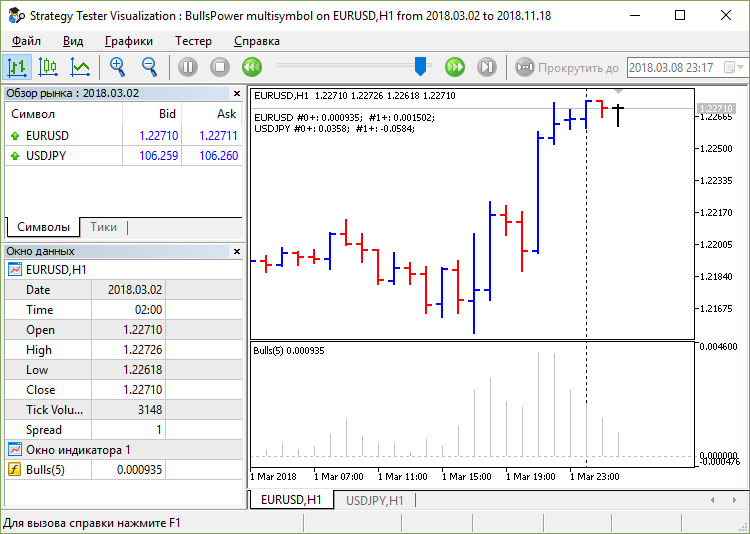Files: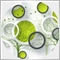56

Is it possible moving the handle part in OnInit() section into OnTick() section ?Moderator
270651

budiali:
Is it possible moving the handle part in OnInit() section into OnTick() section ?

Handle MUST be created ONLY ONCE. The most convenient place to create a handle is OnInit ().Moderator
270651

### An example of working with the ZigZag indicator

Code: ZigZag Example.mq5

Pay attention to the extremum search algorithm: if the value in the indicator buffer is not equal to "0.0" and not equal to "PLOT_EMPTY_VALUE" - it means that We have detected an extremum.

The extremum search goes to " ZigZag: how many candles to check back " bars.

Algorithm of work standard: ONCE in OnInit () we create an indicator.

```//---
int   handle_iCustom;                  // variable for storing the handle of the iCustom indicator
//+------------------------------------------------------------------+
//| Expert initialization function                                   |
//+------------------------------------------------------------------+
int OnInit()
{
//--- create handle of the indicator iCustom
handle_iCustom=iCustom(Symbol(),Period(),"Examples\\ZigZag",InpDepth,InpDeviation,InptBackstep);
//--- if the handle is not created
if(handle_iCustom==INVALID_HANDLE)
{
//--- tell about the failure and output the error code
PrintFormat("Failed to create handle of the iCustom indicator for the symbol %s/%s, error code %d",
Symbol(),
EnumToString(Period()),
GetLastError());
//--- the indicator is stopped early
return(INIT_FAILED);
}
//---
return(INIT_SUCCEEDED);
}```

Next in OnTick (), we make a copy of the indicator data, while using the indicator handle.

```//+------------------------------------------------------------------+
//| Expert tick function                                             |
//+------------------------------------------------------------------+
void OnTick()
{
static long counter=0;
counter++;
if(counter>=15)
counter=0;
else
return;
//---
double ZigzagBuffer[];
ArraySetAsSeries(ZigzagBuffer,true);
int start_pos=0,count=InpCandlesCheck+1;
if(!iGetArray(handle_iCustom,0,start_pos,count,ZigzagBuffer))
return;

string text="";
for(int i=0;i<count;i++)
{
if(ZigzagBuffer[i]!=PLOT_EMPTY_VALUE && ZigzagBuffer[i]!=0.0)
text=text+"\n"+IntegerToString(i)+": "+DoubleToString(ZigzagBuffer[i],Digits());
}
Comment(text);
}```

The function by which the data is copied indicator:

```//+------------------------------------------------------------------+
//| Get value of buffers                                             |
//+------------------------------------------------------------------+
double iGetArray(const int handle,const int buffer,const int start_pos,const int count,double &arr_buffer[])
{
bool result=true;
if(!ArrayIsDynamic(arr_buffer))
{
Print("This a no dynamic array!");
return(false);
}
ArrayFree(arr_buffer);
//--- reset error code
ResetLastError();
//--- fill a part of the iBands array with values from the indicator buffer
int copied=CopyBuffer(handle,buffer,start_pos,count,arr_buffer);
if(copied!=count)
{
//--- if the copying fails, tell the error code
PrintFormat("Failed to copy data from the indicator, error code %d",GetLastError());
//--- quit with zero result - it means that the indicator is considered as not calculated
return(false);
}
return(result);
}```

Result of work: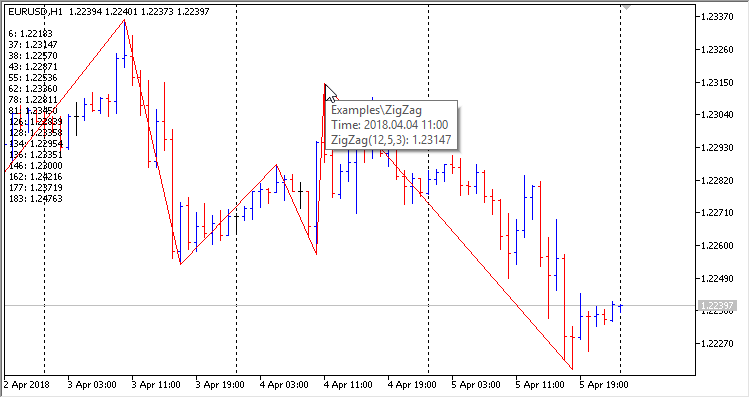Files:Moderator
270651

An example of working with an OBJ_HLINE graphic object.

Advisor OBJ_HLINE follows price has two parameters: Indent up - indent up from the current price and Indent down - indent down from the top line: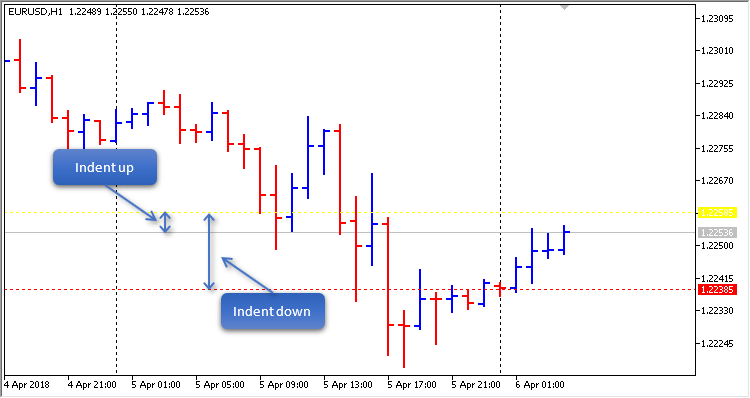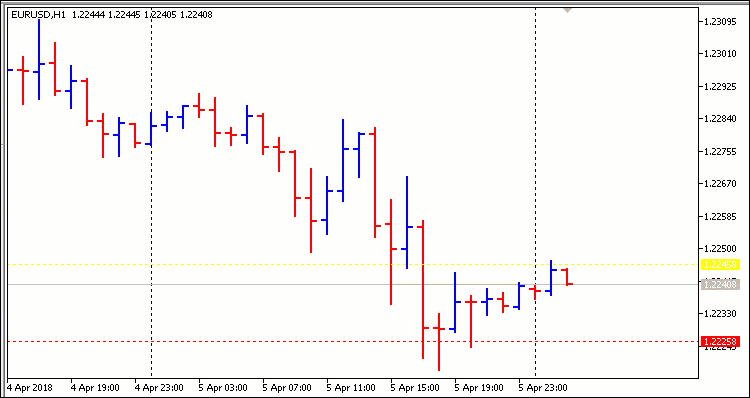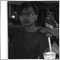123

my idea to make EA without indicators, just rely on candles and tick movements. so at the beginning of the formation of a new candle, EA will analyze and calculate the tick of the opening price. as many as N_Tick we analyze whether the position is located. if within 10 ticks of the candle formed below the close price, sell if opposite, then buy.

I am beginner at mql programming and am making the code I only have a few problems I encountered, the program for reading ticks does not run completely. please help if anyone can correct the program that I made.

thanks.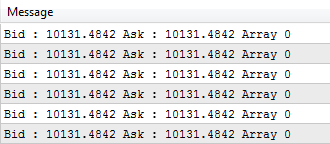```void OnTick()
{
scanTicks();

}

bool NewBar(void)
{
bool iNewBar=false;
static double currPeriodProgress=0;

double lastPeriodProgress=MathMod(TimeCurrent(),PeriodSeconds());

if(lastPeriodProgress<currPeriodProgress)
iNewBar=true;
currPeriodProgress=lastPeriodProgress;
return(iNewBar);
}

void scanTicks()
{
NewBar();

double waktuSekarang;

if(NewBar()==true)
{
waktuSekarang=MathMod(TimeCurrent(),PeriodSeconds());
}

MqlTick tick_array[];

int copied=CopyTicks(_Symbol,tick_array,COPY_TICKS_ALL,0,1);
// Latest loop
for(int i=copied-1; i>=0;i--)
{
MqlTick tick = tick_array[i];

//debug
Print("Bid : ", tick.bid, " Ask : ", tick.ask, " Array ", i);
}
}```
Files:Moderator
270651

Example:

```//+------------------------------------------------------------------+
//|                                                         Temp.mq5 |
//|                                           http://wmua.ru/slesar/ |
//+------------------------------------------------------------------+
#property version   "1.00"
//+------------------------------------------------------------------+
//| Enum Copy Ticks Flags                                            |
//+------------------------------------------------------------------+
enum ENUM_COPY_TICKS_FLAGS
{
info=0,     // COPY_TICKS_INFO
all=2,      // COPY_TICKS_ALL
};
//--- input parameters
input ENUM_COPY_TICKS_FLAGS   InpFlags = trade; // The Copy Ticks Flags:
input uint                    InpCount = 10;    // The number of ticks that you want to receive
//---
datetime ExtPrevBars=0;        // "0" -> D'1970.01.01 00:00';
//+------------------------------------------------------------------+
//| Expert initialization function                                   |
//+------------------------------------------------------------------+
int OnInit()
{
//---

//---
return(INIT_SUCCEEDED);
}
//+------------------------------------------------------------------+
//| Expert deinitialization function                                 |
//+------------------------------------------------------------------+
void OnDeinit(const int reason)
{
//---

}
//+------------------------------------------------------------------+
//| Expert tick function                                             |
//+------------------------------------------------------------------+
void OnTick()
{
//--- we work only at the time of the birth of new bar
datetime time_0=iTime(Symbol(),Period(),0);
if(time_0==ExtPrevBars)
return;
ExtPrevBars=time_0;
//---
MqlTick tick_array[];   // Tick receiving array
//--- Measuring start time before receiving the ticks
uint start=GetTickCount();
//--- Requesting the tick history since 1970.01.01 00:00.001 (parameter from=1 ms)
{
//--- Showing information about the number of ticks and spent time
//--- If the tick history is synchronized, the error code is equal to zero
if(GetLastError()==0)
{
for(int i=0;i<limit;i++)
}
else
PrintFormat("%s: Ticks are not synchronized yet, %d ticks received for %d ms. Error=%d",
}
}
//+------------------------------------------------------------------+
```

Result:

```2019.08.18 09:29:02.686 2019.03.11 02:00:00   EURUSD: received 9 ticks in 0 ms
2019.08.18 09:29:02.686 2019.03.11 02:00:00   Bid : 1.12343 Ask : 1.1236 Array 0
2019.08.18 09:29:02.686 2019.03.11 02:00:00   Bid : 1.12344 Ask : 1.12361 Array 1
2019.08.18 09:29:02.686 2019.03.11 02:00:00   Bid : 1.12342 Ask : 1.12359 Array 2
2019.08.18 09:29:02.686 2019.03.11 02:00:00   Bid : 1.12343 Ask : 1.1236 Array 3
2019.08.18 09:29:02.686 2019.03.11 02:00:00   Bid : 1.12341 Ask : 1.12358 Array 4
2019.08.18 09:29:02.686 2019.03.11 02:00:00   Bid : 1.12342 Ask : 1.12359 Array 5
2019.08.18 09:29:02.686 2019.03.11 02:00:00   Bid : 1.1234 Ask : 1.12357 Array 6
2019.08.18 09:29:02.686 2019.03.11 02:00:00   Bid : 1.12341 Ask : 1.12358 Array 7
2019.08.18 09:29:02.686 2019.03.11 02:00:00   Bid : 1.1234 Ask : 1.12357 Array 8
```
Files:
Temp.mq5  7 kbModerator
32153

Anggono Utomo:

Do not double post!

I have deleted your duplicate post.Moderator
270651

Keith Watford :

Do not double post!

I have deleted your duplicate post.

Nobody did a double post. I did a post transfer from one topic to another: